# How to use the GAMMA function in Excel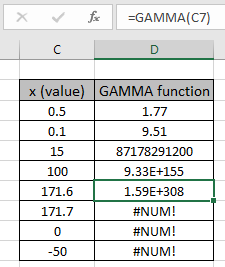In this article, we will learn How to use the GAMMA function in Excel.

What is the GAMMA function?

The gamma distribution is a two-parameter family of continuous probability distributions. The gamma distribution is the maximum entropy probability distribution. For example if you went to the station and to travel you need to buy the ticket. Ticket counter has a queue with a couple of people. Now given some fixed parameters, can you find the probability that you wait more than 5 minutes in the queue? Gamma distribution is used to predict the wait time until the k-th event. Now let's see how the parameters affect the probability factor. Gamma distribution(CDF) can be carried out in two types one is cumulative distribution function, the mathematical representation is given below.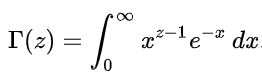Now we proceed to the other part which is probability density function which is extracted when you differentiate the above equation with respect to time. We get the below equation.

The red font part indicated in the denominator is basically the gamma factor. The gamma distribution plot is shown below.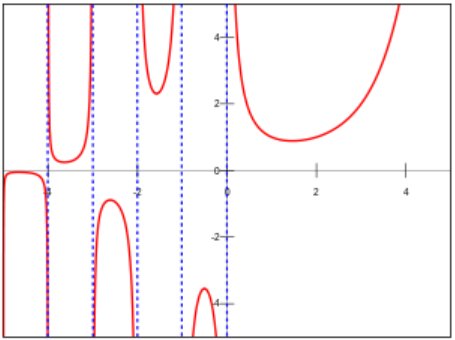As you can see these are complex functions to extract probability using it in excel. So excel provides the inbuilt statistical function to get gamma function results. Let's understand how to use the GAMMA function with an example.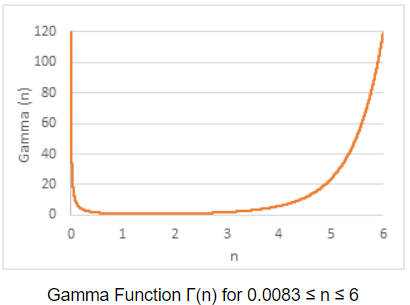GAMMA Function in Excel

The GAMMA function returns the gamma value . It takes the value and the two parameters named alpha and beta. Let's understand these arguments as explained below.

GAMMA Function syntax:

 =GAMMA(x)

x : value at which you want to evaluate.

Example :

All of these might be confusing to understand. Let's understand how to use the function using an example. The Gamma Distribution is frequently used to provide probabilities for sets of values that may have a skewed distribution, such as queuing analysis. For example Waiting in a queue having 2 persons. The service times alpha and beta are independent with a mean of 2 minutes on each person. (Thus the mean service rate is .5/minute). Can you find the probability of waiting more than 5 minutes in the queue?

Use the formula:

 =GAMMA(C3)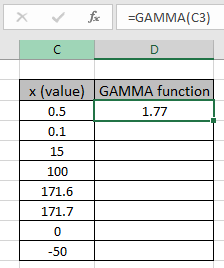As you can see there are very less chances of waiting in a queue for more than 5 minutes which is approximate 10%. I can wait for that period.

Now use the formula to find the probability density distribution.

Use the formula:

 =GAMMA(B3,B4,B5,TRUE)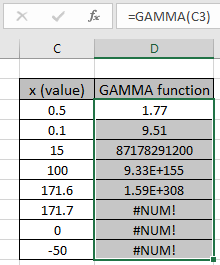As you can see how the new gamma function works in Excel.

Here are all the observational notes using the GAMMA function in Excel
Notes :

1. The function only works with numbers. If any argument other than cumulative is non numeric, the function returns #VALUE! error.
2. The function returns #NUM! Error.
1. If x < = 0
2. If x > 171.7
3. You can feed the arguments to the function directly or using the cell reference as explained in the example.

Hope this article about How to use the GAMMA function in Excel is explanatory. Find more articles on statistical formulas and related Excel functions here. If you liked our blogs, share it with your friends on Facebook. And also you can follow us on Twitter and Facebook. We would love to hear from you, do let us know how we can improve, complement or innovate our work and make it better for you. Write to us at info@exceltip.com.

Related Articles :

How to use Excel GAMMA.DIST Function : Calculate the probability for the cumulative distribution for the pre specified parameters (alpha & beta) using the GAMMA.DIST function in Excel.

How to use Excel NORM.DIST Function : Calculate the Z score for the normal cumulative distribution for the pre specified values using the NORMDIST function in Excel.

How to use Excel NORM.INV Function : Calculate the inverse of Z score for the normal cumulative distribution for the pre-specified probability values using the NORM.INV function in Excel.

How to Calculate Standard Deviation in Excel : To calculate the standard deviation we have different functions in Excel. The standard deviation is the square root of the variance value but It tells more about the dataset than variance.

Regressions Analysis in Excel : Regression is an Analysis Tool, which we use for analyzing large amounts of data and making forecasts and predictions in Microsoft Excel.

How to Create Standard Deviation Graph : The standard deviation tells how much data is clustered around the average of the data. Learn how to create a standard deviation graph here.

How to use the VAR function in Excel : Calculate the variance for the sample dataset in excel using the VAR function in Excel.

Popular Articles :

How to use the IF Function in Excel : The IF statement in Excel checks the condition and returns a specific value if the condition is TRUE or returns another specific value if FALSE.

How to use the VLOOKUP Function in Excel : This is one of the most used and popular functions of excel that is used to lookup value from different ranges and sheets.

How to use the SUMIF Function in Excel : This is another dashboard essential function. This helps you sum up values on specific conditions.

How to use the COUNTIF Function in Excel : Count values with conditions using this amazing function. You don't need to filter your data to count specific values. Countif function is essential to prepare your dashboard.

How to Calculate Standard Deviation in Excel : To calculate the standard deviation we have different functions in Excel. The standard deviation is the square root of the variance value but It tells more about the dataset than variance.

Terms and Conditions of use

The applications/code on this site are distributed as is and without warranties or liability. In no event shall the owner of the copyrights, or the authors of the applications/code be liable for any loss of profit, any problems or any damage resulting from the use or evaluation of the applications/code.